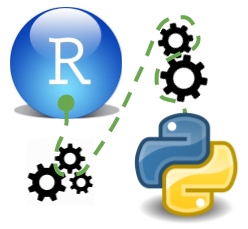# Automated Machine Learning

We have seen in the previous post on advanced scikitlearn methods that using pipes in `scikitlearn` allows us to write pretty generalizable code, however we still need to customize our modelling pipeline to the algorithms that we want to use. However theoretically since `scikitlearn` uses a unified synthax there is no reason why we should not try all the modelling algorithms supported by scikitlearn. We can also combine the modelling algorithm with various feature selection and preprocessing steps. The first limiting factor is that theoretically we need to define the hyperparameter space that is being searched for each model even if we are using randomized search. Which requires some manual coding the second limiting factor is computational power it will simply take a very long time to go through all possible combinations of pipes that we could build.

Packages for automated machine learning have taken care of the manual work we would need to do to program the hyperparameter search and mitigate the problem of computational power by employing specific search strategies that allow us to preselect pipes that are likely to succeed and optimise hyperparameter search in a way that we do not have to test every single combinations.

## `tpot`

`tpot` is a data science assistant that iteratively constructs `sklearn` pipelines and optimises them using genetic programming algorithms that are able to optimize multiple criteria simulaneously while minimizing complexity at the same time. It uses a package called deap

• Supports regression and classification
• Supports the usual performance metrics
• Is meant to run for hours to days
• We can inspect the process anytime and look at intermediate results
• We can limit algorithms and hyperparameter space (not so usefull at the moment because we have to sepcifiy the whole pyrameter range and basically get stuck doing grid search)
• `tpot` can generate python code to reconstruct the best models

We have prepared the data that we are going to use in the previous post on advanced scikitlearn methods. It is basically the titanic dataset with imputed numerical and categorical variables.

``````import feather

y = df['y']
X = df.drop('y', axis = 1)\
.as_matrix()
``````
``````C:\anaconda\envs\py36r343\lib\site-packages\ipykernel\__main__.py:6: FutureWarning: Method .as_matrix will be removed in a future version. Use .values instead.
``````

### Run

We are using a limited version of `tpot` which uses only fast algorithms

``````from tpot import TPOTClassifier

pipeline_optimizer = TPOTClassifier(generations=2
, population_size=2
, offspring_size = 5
## TPOT will evaluate population_size
## + generations × offspring_size = pipelines
## in total.
, cv=5
, random_state=42 ##seed
, verbosity=2 ## print progressbar
, n_jobs = 4
, warm_start = True ## allows us to restart
, scoring = 'roc_auc'
, config_dict = 'TPOT light' ## only uses fast algorithms
)

pipeline_optimizer.fit(X,y)
``````
``````HBox(children=(IntProgress(value=0, description='Optimization Progress', max=12), HTML(value='')))

Generation 1 - Current best internal CV score: 0.8616010162631975
Generation 2 - Current best internal CV score: 0.8628532835333793

Best pipeline: LogisticRegression(Normalizer(input_matrix, norm=l1), C=20.0, dual=False, penalty=l1)

TPOTClassifier(config_dict='TPOT light', crossover_rate=0.1, cv=5,
disable_update_check=False, early_stop=None, generations=2,
max_eval_time_mins=5, max_time_mins=None, memory=None,
mutation_rate=0.9, n_jobs=4, offspring_size=5,
periodic_checkpoint_folder=None, population_size=2,
verbosity=2, warm_start=True)
``````
``````pipeline_optimizer.score(X,y)
``````
``````0.8745406320902439
``````

### Export best modelling pipeline as python code

``````pipeline_optimizer.export('./data/pipe.py')
``````
``````True
``````

``````pipeline_optimizer.fitted_pipeline_
``````
``````Pipeline(memory=None,
steps=[('normalizer', Normalizer(copy=True, norm='l1')), ('logisticregression', LogisticRegression(C=20.0, class_weight=None, dual=False, fit_intercept=True,
intercept_scaling=1, max_iter=100, multi_class='ovr', n_jobs=1,
penalty='l1', random_state=None, solver='liblinear', tol=0.0001,
verbose=0, warm_start=False))])
``````

### Get all tested pipes

``````pipeline_optimizer.evaluated_individuals_
``````
``````{'DecisionTreeClassifier(input_matrix, DecisionTreeClassifier__criterion=entropy, DecisionTreeClassifier__max_depth=2, DecisionTreeClassifier__min_samples_leaf=12, DecisionTreeClassifier__min_samples_split=4)': {'crossover_count': 0,
'generation': 'INVALID',
'internal_cv_score': 0.8557279030479364,
'mutation_count': 2,
'operator_count': 1,
'predecessor': ('DecisionTreeClassifier(input_matrix, DecisionTreeClassifier__criterion=entropy, DecisionTreeClassifier__max_depth=3, DecisionTreeClassifier__min_samples_leaf=12, DecisionTreeClassifier__min_samples_split=4)',)},
'DecisionTreeClassifier(input_matrix, DecisionTreeClassifier__criterion=entropy, DecisionTreeClassifier__max_depth=3, DecisionTreeClassifier__min_samples_leaf=12, DecisionTreeClassifier__min_samples_split=4)': {'crossover_count': 0,
'generation': 'INVALID',
'internal_cv_score': 0.8616010162631975,
'mutation_count': 1,
'operator_count': 1,
'predecessor': ('GaussianNB(input_matrix)',)},
'GaussianNB(input_matrix)': {'crossover_count': 0,
'generation': 0,
'internal_cv_score': 0.816504621285001,
'mutation_count': 0,
'operator_count': 1,
'predecessor': ('ROOT',)},
'KNeighborsClassifier(BernoulliNB(input_matrix, BernoulliNB__alpha=10.0, BernoulliNB__fit_prior=True), KNeighborsClassifier__n_neighbors=21, KNeighborsClassifier__p=1, KNeighborsClassifier__weights=distance)': {'crossover_count': 0,
'generation': 'INVALID',
'internal_cv_score': 0.8499987059406567,
'mutation_count': 1,
'operator_count': 2,
'predecessor': ('KNeighborsClassifier(input_matrix, KNeighborsClassifier__n_neighbors=21, KNeighborsClassifier__p=1, KNeighborsClassifier__weights=distance)',)},
'KNeighborsClassifier(BernoulliNB(input_matrix, BernoulliNB__alpha=100.0, BernoulliNB__fit_prior=True), KNeighborsClassifier__n_neighbors=21, KNeighborsClassifier__p=1, KNeighborsClassifier__weights=distance)': {'crossover_count': 0,
'generation': 'INVALID',
'internal_cv_score': 0.8484891857167135,
'mutation_count': 1,
'operator_count': 2,
'predecessor': ('KNeighborsClassifier(input_matrix, KNeighborsClassifier__n_neighbors=21, KNeighborsClassifier__p=1, KNeighborsClassifier__weights=distance)',)},
'KNeighborsClassifier(input_matrix, KNeighborsClassifier__n_neighbors=21, KNeighborsClassifier__p=1, KNeighborsClassifier__weights=distance)': {'crossover_count': 0,
'generation': 0,
'internal_cv_score': 0.8470927552585381,
'mutation_count': 0,
'operator_count': 1,
'predecessor': ('ROOT',)},
'KNeighborsClassifier(input_matrix, KNeighborsClassifier__n_neighbors=22, KNeighborsClassifier__p=1, KNeighborsClassifier__weights=distance)': {'crossover_count': 0,
'generation': 'INVALID',
'internal_cv_score': 0.846680654239431,
'mutation_count': 1,
'operator_count': 1,
'predecessor': ('KNeighborsClassifier(input_matrix, KNeighborsClassifier__n_neighbors=21, KNeighborsClassifier__p=1, KNeighborsClassifier__weights=distance)',)},
'LogisticRegression(MinMaxScaler(input_matrix), LogisticRegression__C=20.0, LogisticRegression__dual=False, LogisticRegression__penalty=l1)': {'crossover_count': 0,
'generation': 'INVALID',
'internal_cv_score': 0.8584054700315054,
'mutation_count': 2,
'operator_count': 2,
'predecessor': ('LogisticRegression(input_matrix, LogisticRegression__C=20.0, LogisticRegression__dual=False, LogisticRegression__penalty=l1)',)},
'LogisticRegression(Normalizer(input_matrix, Normalizer__norm=l1), LogisticRegression__C=20.0, LogisticRegression__dual=False, LogisticRegression__penalty=l1)': {'crossover_count': 0,
'generation': 'INVALID',
'internal_cv_score': 0.8628532835333793,
'mutation_count': 2,
'operator_count': 2,
'predecessor': ('LogisticRegression(input_matrix, LogisticRegression__C=20.0, LogisticRegression__dual=False, LogisticRegression__penalty=l1)',)},
'LogisticRegression(input_matrix, LogisticRegression__C=20.0, LogisticRegression__dual=False, LogisticRegression__penalty=l1)': {'crossover_count': 0,
'generation': 'INVALID',
'internal_cv_score': 0.8584323502037432,
'mutation_count': 1,
'operator_count': 1,
'predecessor': ('GaussianNB(input_matrix)',)},
'LogisticRegression(input_matrix, LogisticRegression__C=20.0, LogisticRegression__dual=False, LogisticRegression__penalty=l2)': {'crossover_count': 0,
'generation': 'INVALID',
'internal_cv_score': 0.8586414616613588,
'mutation_count': 2,
'operator_count': 1,
'predecessor': ('LogisticRegression(input_matrix, LogisticRegression__C=20.0, LogisticRegression__dual=False, LogisticRegression__penalty=l1)',)}}
``````

## `auto-sklearn`

`auto-skelarn` uses bayesian methods to optimize computing time. We will add an example in a later version of this post

## Summary

Even though auto-sklearn still needs to be tested we could already obtain pretty decent results using `tpot` with a ROC score of 0.87 which is higher then our previous attempts. Normally I would follow the following strategy to select a model.

• Test all models on the same cv pairs
• Calculate mean and SEM for the performance metric of each variant
• Look at the model with the lowest mean
• Select the simplest model whose mean is still in the range of the overall lowest mean + SEM

However `tpot` does not give us the SEM values thus we cannot select the model which it presents us to be the best and compare it to simpler ones it might have fitted. Given that the `tpot` algorithm is already minimizing the complexity we should simply accept the best model it returns. We should however then compare it to simpler models we can come up with to have a frame of reference to compare it to and of course we should check the `tpot` model for plausibility.